The Crystal Reference System edit page

Commonly the crystal coordinate system is defined by the crystallographic axes $$\vec a$$, $$\vec b$$, $$\vec c$$, The length [a,b,c] and the angles [alpha,beta,gamma] between theses axes needs to be specified when defining a variable of type crystalSymmetry.

## Need of a Euclidean reference system

However, there are many crystal properties, like orientation or tensorial properties, that are described with respect to an Euclidean reference system $$\vec x$$, $$\vec y$$, $$\vec z$$ as oposed to the crystallographic axes $$\vec a$$, $$\vec b$$s, $$\vec c$$. Most importantly, Euler angles describe orientations as subsequent rotations about the $$\vec z$$, $$\vec x$$ and $$\vec z$$ axis. Hence, we need to inscribe an Eucledian reference system $$\vec x$$, $$\vec y$$, $$\vec z$$ into the crystallogaphic reference system $$\vec a$$, $$\vec b$$, $$\vec c$$.

Note, that also the allignment of the crystal axes $$\vec a$$, $$\vec b$$ and $$\vec c$$ with respect to the atomic lattice, and hence it symmetries, follows different conventions. These are discussed in the section Alignment of the Crystal Axes.

## Cubic, tetragona and orthorhombic symmetries

In orthorhombic, tetragonal and cubic crystal symmetry the crystal reference system $$\vec a$$, $$\vec b$$, $$\vec c$$ is itsself an Euclidean one and, hence, setting $$\vec x$$ parallel to $$\vec a$$, $$\vec y$$ parallel to $$\vec b$$ and $$\vec z$$ parallel to $$\vec c$$ is a canonical choice.

As for such symmetries this is also the default in MTEX there is no need to specify the alignment seperately.

## Trigonal and hexagonal materials

For trigonal and hexagonal materials the z axis is commonly aligned with the $$\vec c$$ axis. As for the $$\vec x$$ and $$\vec y$$ axes they are either aligned with the $$\vec a$$ or $$\vec b$$ axes.

The following command alignes the $$\vec x$$ axes to the $$\vec a$$ axes and the $$\vec z$$ axes to the $$\vec c$$ axes.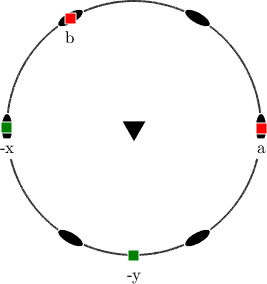In contrast the following command alignes the $$\vec y$$ axes to the $$\vec a$$ axes and the $$\vec z$$ axes to the $$\vec c$$ axes.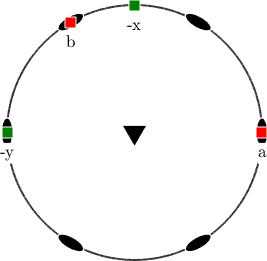The only difference between the above two plots is the position of the $$\vec x$$ and $$\vec y$$ axes. The reason is that visualizations relative to the crystal reference system, e.g., inverse pole figures, are in MTEX aligned on the screen according to the b-axis.

This on secreen alignment can be easily modified by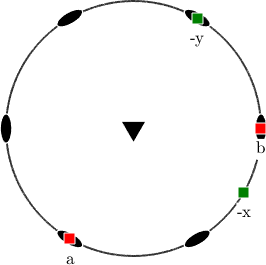It should be stressed that the alignment between the Eucledean crystal axes $$\vec x$$, $$\vec y$$, $$\vec z$$ and the crystallographic axes $$\vec a$$, $$\vec b$$ and $$\vec c$$ is crucial for many computations. The difference between both setups becomes more vsible if we plot crystal shapes in the $$\vec x$$, $$\vec y$$, $$\vec z$$ coordinate system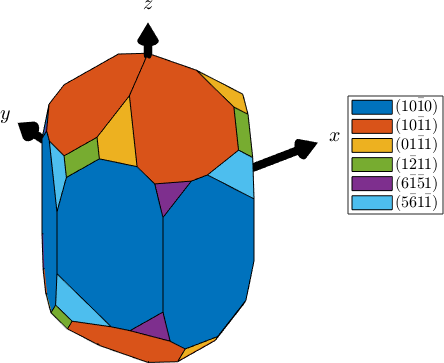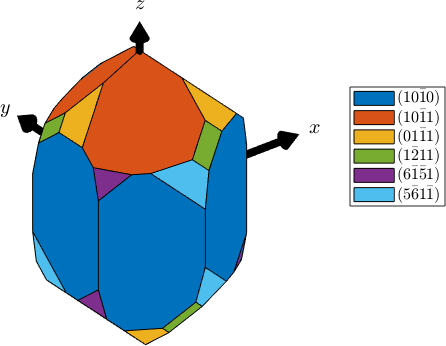Most important is the difference if Euler angles are used to describe orientation. Lets consider the following two orientations

and visualize them in a pole figure.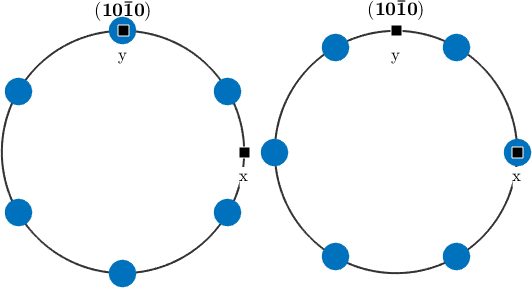We observe that both pole figures are rotated with respect to each other by 30 degree. Indeed computing the misorientation angle between both orientations gives us

In many cases MTEX automatically recognizes different setups and corrects for this. In order to manually transform orientations or tensors from one reference frame into another reference frame one might use the command transformReferenceFrame. The following command transfroms the reference frame of orientation ori_y2a into the reference frame cs_x2a

## Triclinic and monoclinic symmetries

In triclinic and monoclinic symmetries even more different setups are used. As two perpedicular crystal axes are required to align with $$\vec x$$, $$\vec y$$ or $$\vec z$$ one ussually chooses one crystal axis from the direct coordinate system, i.e., $$\vec a$$, $$\vec b$$ or $$\vec c$$, and the second crystal axis from the reciprocal axes $$\vec a^*$$, $$\vec b^*$$ or $$\vec c^*$$. Typical examples for such setups are

or